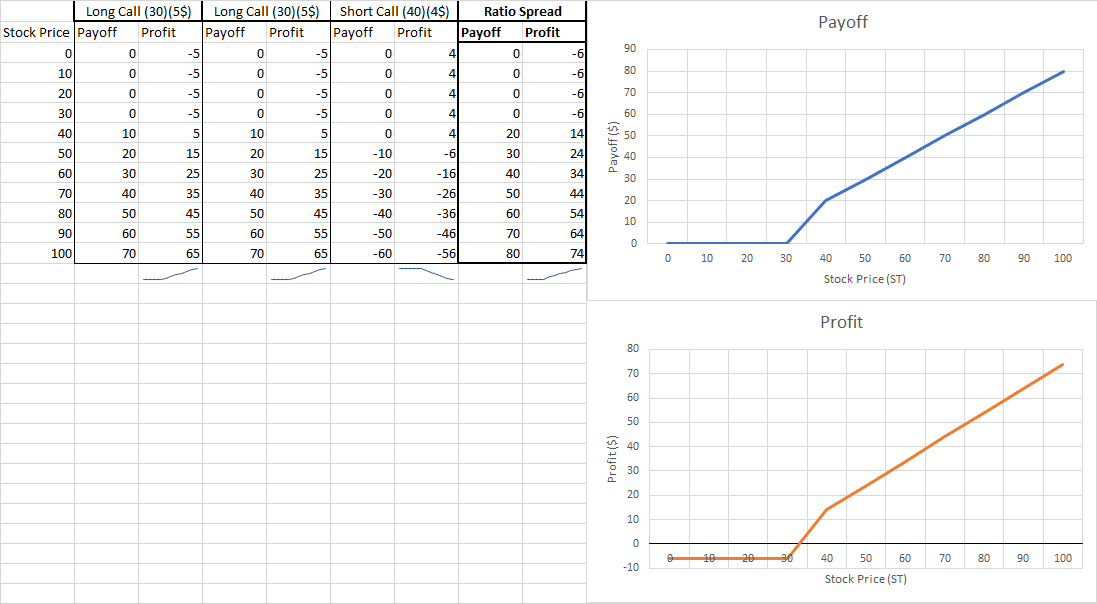# Ratio Strategy

A ratio is an option strategy that is created by having X amount of call options at Strike Price 1 and shorting Y amount of call options at Strike Price 2. By creating a ratio, you are creating an option strategy where you can reduce your total option costs by shorting more call options are a higher strike price.

## What are its components?

A ratio strategy has four components:

• X amount of long call options
• Y amount of short call options

## When and why should I have a ratio strategy?

You should have a ratio strategy if you have a bullish view on the performance of the underlying asset. By shorting Y amount of call options, you are consistently reducing your option costs and eventually creating a zero-cost strategy. The ratio can be adjusted based on the investor’s perception of asset. The same concept can be applied with puts.

## What is the payoff and profit graph?## What is the break-even point?

The break-even point of a ratio strategy can be defined by finding the stock price where the bear spread generates a zero-dollar profit. By adding all contracts and equating it to zero, you should solve for ST. There is a possibility where two stock prices can break-even. To find both points, you should create scenarios to define the payoffs. For example, the payoff when ST<30, 30<ST<40, 40<ST.